We present a detailed discussion of our novel diagrammatic coupled cluster Monte Carlo (diagCCMC) [Scott et al. J. Phys. Chem. Lett. 10, 925 (2019)]. The diagCCMC algorithm performs an imaginary-time propagation of the similarity-transformed coupled cluster Schrödinger equation. Imaginary-time updates are computed by the stochastic sampling of the coupled cluster vector function: each term is evaluated as a randomly realized diagram in the connected expansion of the similarity-transformed Hamiltonian. We highlight similarities and differences between deterministic and stochastic linked coupled cluster theory when the latter is re-expressed as a sampling of the diagrammatic expansion and discuss details of our implementation that allow for a walker-less realization of the stochastic sampling. Finally, we demonstrate that in the presence of locality, our algorithm can obtain a fixed errorbar per electron while only requiring an asymptotic computational effort that scales quartically with system size, independent of the truncation level in coupled cluster theory. The algorithm only requires an asymptotic memory cost scaling linearly, as demonstrated previously. These scaling reductions require no ad hoc modifications to the approach.

The goal of quantum chemistry is to provide accurate and cost-effective methodologies for the solution of the molecular electronic Schrödinger equation. One needs to be able not only to reproduce experimentally measurable observables but also to understand the microscopic origin of these measurements and eventually predict and guide experiments.

Stochastic approaches to the solution of the Schrödinger equation provide an appealing alternative to deterministic strategies, and a number of Monte Carlo (MC) sampling methods have been continuously developed since the early days of quantum chemistry.1,2 At the cost of introducing statistical uncertainty in the results, quantum Monte Carlo (QMC) offers a low-scaling, parallelizable route to high-accuracy results. Despite the favorable scaling and scalability, QMC suffers from two well-known problems. The statistical errorbar can be decreased but at a very slow rate with increasing length of the simulation, that is, a larger number of random samples. Furthermore, for fermionic systems of interest in molecular electronic structure theory, the nodal structure of the wavefunction needs to be fixed a priori to avoid collapse onto the bosonic ground state,3,4 introducing an uncontrolled approximation that, thus, far cannot be efficiently relaxed to exactness. Despite this, their low polynomial scaling allows large-scale applications, particularly within condensed matter systems where they can provide accurate results while allowing extrapolation to remove finite-size errors.1,2,5

Within the deterministic realm, the two above-mentioned problems do not appear. No statistical uncertainity riddles the results, and the methods are all formulated in the appropriate Fock space, guaranteeing that the solution, while approximate, is properly antisymmetrized. The coupled cluster (CC) wavefunction ansatz arguably provides the most effective framework for accurate simulations of single-reference molecular systems. The CC model provides an exponential parametrization of the molecular electronic wavefunction and enjoys a number of favorable properties. It provides a systematic route toward the exact, full configuration interaction (FCI) solution, while maintaining size-extensivity and size-consistency of results at any truncation level. Despite the exponential, nonlinear parametrization of the wavefunction, the computational cost of CC theory scales as a polynomial of system size, albeit with potentially high values for the exponents. Scaling and scalability are thus much less favorable than with stochastic approaches: a number of approximations have to be introduced,6–27 and many technical challenges need to be surmounted.28–36 In particular, while many high-performance implementations of coupled cluster with single and double (CCSD) substitutions and CCSD with perturbative triples correction [CCSD(T)] are nowadays available, the large gain in efficiency seen in local theories has yet to be reproduced for higher truncation levels in the CC hierarchy.

With these considerations in mind, efforts in the past decade have been directed at combining the best of both worlds into the formulation and implementation of Fock-space QMC methods. The full configuration interaction quantum Monte Carlo (FCIQMC) was the first such method to be presented, and the FCI secular problem is solved as the dynamics of a population of signed particles.37–40 This results in an exponentially scaling algorithm but with a dramatically reduced prefactor. Building on this, some of us have further developed a similar projector MC algorithm to solve the unlinked and linked CC equations.41–45 These CCMC algorithms, implemented in the HANDE-QMC software package,46 are fully general with respect to the excitation level, allowing one to perform arbitrary order CC simulations with a sparse representation of the wavefunction.

We recently showed that neither FCIQMC nor CCMC rigorously fulfills size-extensivity for noninteracting systems.47 Both algorithms perform imaginary-time propagation of unlinked many-body equations,48 which results in the unnecessary sampling of zero-on-average terms. This unnecessary work negatively impacts the memory and central processing unit (CPU) costs of the simulation and is particularly severe for CCMC, as it quickly precludes scaling to larger systems and/or higher orders of CC theory. To remedy this situation, we put forth a MC algorithm that performs the imaginary-time propagation governed by the linked CC equations. These are evaluated by the random sampling of the connected terms in the similarity-transformed Hamiltonian, conveniently represented as a diagrammatic expansion. The diagCCMC algorithm restores size-extensivity, and our preliminary tests have shown how localization can be readily exploited without further assumptions.49

We should note that this is not the only avenue toward leveraging the benefits of MC sampling within the CC approach. Deustua et al. have shown how deterministic iterative CC solvers can be seeded with amplitudes from partially converged Fock-space QMC results. Combined with moment expansion corrections,50–52 this approach is a powerful technique, enabling access to higher levels of CC theory at a reduced cost. However, the high computational scaling of the QMC methods used to determine important higher-level amplitudes will eventually dominate the overall computational cost of this approach, and thus, our work provides a complementary solution.

In this work, we will first describe in detail the theoretical framework in which our diagCCMC algorithm rests. Section II summarizes CC theory with particular emphasis on its diagrammatic formulation. In Sec. III, we present a derivation of the imaginary-time update step and its usage in the CCMC and diagCCMC algorithms. We will then discuss the structure of the implemented algorithm and highlight differences and similarities to a deterministic implementation of CC theory.

We will use the tensor notation for second quantization.53–55 We denote the elementary, anticommuting fermion creation and annihilation operators as:

$âp†=âp≡p^+, âp≡p^−.$
(1)

A k-electron excitation operator with respect to the physical vacuum (|vac⟩) is the product of k creation and k annihilation operators. In tensor notation,

$âr1r2…rks1s2…sk=ŝ1+ŝ2+…ŝk+r^k−…r^2−r^1−,$
(2)

such excitation operators are particle number-conserving. Explicitly, the one- and two-electron substitutions are

$âqp=p^+q^−, ârspq=p^+q^+ŝ−r^−.$
(3)

The Born–Oppenheimer molecular electronic Hamiltonian is then expressed as

$Ĥ=∑pqhpqâqp+12∑pqrsgpqrsârspq=∑pqhpqâqp+14∑pqrsg¯pqrsârspq,$
(4)

where the integrals are given in an orthonormal basis of one-electron spin–orbitals,

$hpq=∫ dxϕp*(x)−12∇2+VeNϕq(x),$
(5a)
$gpqrs=∫ dx∫ dx′ϕp*(x)ϕq*(x′)ϕr(x)ϕs(x′)|r1−r2|=⟨pq|rs⟩,$
(5b)
$g¯pqrs=gpqrs−gpqsr=⟨pq‖rs⟩.$
(5c)

For single-reference theories, it is more convenient to work in terms of the Fermi, rather than the physical, vacuum state. Our Fermi vacuum will be a single-determinant reference function |D0⟩ for an N-electron system with 2M spin–orbitals.

Occupied one-particle states in |D0⟩, i1, i2, …, iN, will be referred to as hole states, whereas virtual one-particle states, aN+1, aN+2, …, will be referred to as particle states. A normal-ordered k-electron substitution operator will be denoted as

$êr1r2…rks1s2…sk.$
(6)

Using Wick’s theorem,55,56 these operators can be rewritten as a finite sum of subsets of permutations of elementary operators times contractions. The latter are elements of k-electron reduced density matrices (k-RDMs),

$γr1r2…rks1s2…sk≡D0|âr1r2…rks1s2…sk|D0.$
(7)

Thus, the normal-ordered one- and two-electron substitutions are57

$êr1s1=âr1s1−γr1s1, êr1r2s1s2=âr1r2s1s2−(γr1s1âr2s2+γr2s2âr1s1−γr2s1âr1s2−γr1s2âr2s1−γr1r2s1s2).$
(8)

Imposing normal ordering on the molecular Hamiltonian, we obtain

$ĤN=F^+Φ^=∑pqfpqêqp+14∑pqrsg¯pqrsêrspq=Ĥ−Eref,$
(9)

where the energy of the reference determinant, the one-body Fock operator, and the two-body fluctuation potential appear,

$Eref=⟨D0|Ĥ|D0⟩=∑ihii+12∑ijg¯ijij,fpq=hpq+∑ig¯piqi.$
(10)

We will use the symbol $τ^k$ for pure excitation operators or excitors. These are k-electron substitutions between hole and particle states in the reference determinant and, thus, particle number- and charge-conserving.

Since the k-RDMs in (7) are zero whenever any of the indices, upper or lower, refers to a particle state, the excitors are automatically normal-ordered,

$τ^k=âi1i2…ika1a2…ak=êi1i2…ika1a2…ak=â1+â2+…âk+ı^k−…ı^2−ı^1−=â1+ı^1−â2+…âk+ı^k−.$
(11)

Here, we have introduced the multi-index $k=a1a2…aki1i2…ik$ to compactly represent the k-electron substitution affected by the excitor, which is, up to a phase, a k-excited determinant,

$|Dk =τ^k|D0 ∝a1a2…aki1i2…ik.$
(12)

A summary of our notation can be found in Table I.

TABLE I.

Overview of notation and nomenclature.

SymbolShort description
|D0⟩ The reference Slater determinant
$âp$ Fermion creation operator
$âp$ Fermion annihilation operator
$âr1r2…rks1s2…sk$ k-electron excitation operator with respect to the physical vacuum
$êr1r2…rks1s2…sk$ k-electron excitation operator, normal-ordered with respect to the reference
j, k, … Replacement multi-indices, e.g., $k=a1a2…aki1i2…ik$
|Dk⟩ The k-th replacement excited determinant
p, q, r, s, … General spin–orbital indices
i1, i2, …, ik Hole spin–orbitals in |D0⟩
a1, a2, …, ak Particle spin–orbitals in |D0⟩
$τ^k$ Excitor for the k-th replacement
tk Cluster amplitude for the k-th replacement
$tkτ^k$ Connected (non-composite) cluster
$12!tktlτ^kτ^l$ Disconnected (composite) cluster
SymbolShort description
|D0⟩ The reference Slater determinant
$âp$ Fermion creation operator
$âp$ Fermion annihilation operator
$âr1r2…rks1s2…sk$ k-electron excitation operator with respect to the physical vacuum
$êr1r2…rks1s2…sk$ k-electron excitation operator, normal-ordered with respect to the reference
j, k, … Replacement multi-indices, e.g., $k=a1a2…aki1i2…ik$
|Dk⟩ The k-th replacement excited determinant
p, q, r, s, … General spin–orbital indices
i1, i2, …, ik Hole spin–orbitals in |D0⟩
a1, a2, …, ak Particle spin–orbitals in |D0⟩
$τ^k$ Excitor for the k-th replacement
tk Cluster amplitude for the k-th replacement
$tkτ^k$ Connected (non-composite) cluster
$12!tktlτ^kτ^l$ Disconnected (composite) cluster

The coupled cluster wavefunction is parametrized as an exponential transformation of a reference single-determinant wavefunction |D0⟩,

$|CC =expT^|D0 ,$
(13)

where the cluster operator $T^$ is given as a sum of second-quantized excitation operators,

$T^=∑mT^m,$
(14)

with the mth order cluster operators expressed as sums of excitors weighted by the corresponding cluster amplitudes,

$T^m=∑k∈mthreplacementstkτ^k=1(k!)2∑a1,a2,…,aki1,i2,…,ikta1a2…aki1i2…ikêi1i2…ika1a2…ak.$
(15)

Note that in the tensor notation adopted, upper and lower indices of the cluster amplitudes appear reversed with respect to other conventions.

The CC correlation energy is the right eigenvalue of the Schrödinger equation for the normal-ordered Hamiltonian in (9),

$ĤN|CC =ΔECC|CC .$
(16)

This equation is solved by performing a similarity transformation of the Hamiltonian,

$exp−T^Ĥ⁡expT^|D0 =H¯|D0 =ΔECC|D0 ,$
(17)

and then projecting onto the excitation manifold {|Dj⟩},

$⟨D0|H¯|D0⟩=ΔECC,$
(18a)
$⟨Dk|H¯|D0⟩=ωk(t).$
(18b)

Equation (18b) defines the CC residual ωk(t), which is zero at a solution of the nonlinear linked equations.58 Whereas (16) and (18a) can be proved to be identical,59 the linked formulation in the latter is size-extensive order-by-order and term-by-term. For notational convenience, we have dropped the subscript N for the Hamiltonian. The similarity-transformed Hamiltonian $H¯$ can be expanded into a Baker–Campbell–Hausdorff (BCH) commutator series,

$exp−T^Ĥ⁡expT^=H¯=∑n≥01n!(ĤT^̲n), (ĤT^̲)=def[Ĥ,T^].$
(19)

For the molecular Hamiltonian in Eq. (4), at most two-body operators are involved. Hence, regardless of the truncation level in the cluster operator $T^$, the expansion truncates at the fourfold nested commutator,

$H¯=∑n=041n!(ĤT^̲n)=Ĥ+[Ĥ,T^]+12![[Ĥ,T^],T^] + 13![[[Ĥ,T^],T^],T^]+14![[[[Ĥ,T^],T^],T^],T^],$
(20)

showing that only finitely many terms are included in Eq. (18b). Despite the fact that $H¯$ is no longer Hermitian, the linked formulation is still more advantageous than the unlinked formulation.59–61

Since all excitors are normal-ordered and commuting, Wick’s theorem56,60,61 lets us reduce the Hamiltonian-excitor products to only those terms that are connected (in the diagrammatic sense). Excitors will only appear to the right of the Hamiltonian, and only terms where each excitor shares at least one index with the Hamiltonian will be nonzero,

$H¯=(Ĥ⁡expT^)c=Ĥ+(ĤT^)c+12!(ĤT^T^)c+13!(ĤT^T^T^)c + 14!(ĤT^T^T^T^)c.$
(21)

The requirement of shared indices between the Hamiltonian and cluster coefficients enables the resulting equations to be solved via a series of tensor contractions: a process highly amenable to rapid evaluation on conventional computing architectures62 but non-trivial to parallelize.28,29,35

The algebraic derivation of the linked CC equations to a general truncation level from Eq. (18b) is lengthy and error prone. A diagrammatic representation can be effectively used to generate all unique terms in the equations.60,63,64 The normal-ordering and application of Wick’s theorem are key to these developments. The normal-ordered Hamiltonian features 13 interaction vertices, 4 coming from the Fock operator,

$F=∑a1a2fa1a2êa2a1+∑i1i2fi1i2êi2i1+∑i1a1fi1a1êa1i1+∑a1i1fa1i1êi1a1,$
(22)

and 9 from the fluctuation potential,

$Φ=14∑a1a2a3a4g¯a1a2a3a4êa3a4a1a2+14∑i1i2i3i4g¯i1i2i3i4êi3i4i1i2+∑a1i1a2i2g¯a1i1a2i2êa2i2a1i1 + 12∑a1a2a3i1g¯a1a2a3i1êa3i1a1a2+12∑i1a1i2i3g¯i1a1i2i3êi2i3i1a1+12∑a1i1a2a3g¯a1i1a2a3êa2a3a1i1 + 12∑i1i2i3a1g¯i1i2i3a1êi3a1i1i2+14∑i1i2a1a2g¯i1i2a1a2êa1a2i1i2+14∑a1a2i1i2g¯a1a2i1i2êi1i2a1a2.$
(23)

Each of these vertices can be characterized by an integer representing their excitation level (0, ±1, ±2) and by a sign sequence encoding the pattern of open particle (+) and hole (−) lines below the interaction vertex (see Table II). Cluster operators can be classified similarly in terms of their excitation level (any integer ≥1) and their sign sequence.

TABLE II.

The thirteen interaction vertices of the normal-ordered Hamiltonian with the corresponding matrix elements, excitation levels, and Kucharski–Bartlett sign sequences.63

VertexMatrix elementExcitation levelSign sequence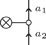$fa1a2$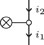$fi1i2$ −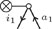$fi1a1$ −1 +−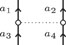$g¯a1a2a3a4$ ++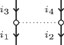$g¯i1i2i3i4$ −−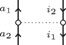$g¯a1i1a2i2$ +−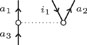$g¯a1a2a3i1$ +1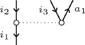$g¯i1a1i2i3$ +1 ++−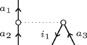$g¯a1i1a2a3$ −1 −
10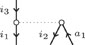$g¯i1i2i3a1$ −1 +−−
11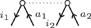$g¯i1i2a1a2$ −2 ++−−
12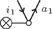$fa1i1$ +1
13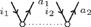$g¯a1a2i1i2$ +2
VertexMatrix elementExcitation levelSign sequence$fa1a2$$fi1i2$ −$fi1a1$ −1 +−$g¯a1a2a3a4$ ++$g¯i1i2i3i4$ −−$g¯a1i1a2i2$ +−$g¯a1a2a3i1$ +1$g¯i1a1i2i3$ +1 ++−$g¯a1i1a2a3$ −1 −
10$g¯i1i2i3a1$ −1 +−−
11$g¯i1i2a1a2$ −2 ++−−
12$fa1i1$ +1
13$g¯a1a2i1i2$ +2

For any given excitation level in the allowed manifold (up to double excitations for CCSD, triple excitations for CCSDT, and so forth), the diagrammatic generation of the corresponding CC equations proceeds via the following steps:

1. At the bottom, we draw a combination of at most four excitors.

2. At the top, we draw a Hamiltonian vertex. The valid vertices are limited by two requirements: (a) the final diagram be connected and (b) the overall excitation level of the projection manifold.

3. We pair the Hamiltonian vertex and excitor(s) sign sequences in all distinct ways to generate the sign sequences for all unique diagrams. The sign sequence encodes the diagram topology and ensuing contraction pattern.

4. We read the algebraic expression for the corresponding term in the CC equations off from the generated diagrams. The rules of interpretation associate target indices to the external (open) lines and dummy summation indices to the internal lines, Hamiltonian matrix elements to the interaction vertices, and products of amplitudes to the excitor vertices. Topological and permutational symmetries are taken into account by similar simple rules.60,61,63

The rules for generating and interpreting diagrams as algebraic expressions are independent of the CC truncation order and can be encoded into a computer program.61,65–70 However, a proper factorization of intermediates is essential to achieve acceptable time to solution and memory requirements.66

The solution of the CC equations can be achieved by means of stochastic algorithms. This stochastic realization is, however, not unique, and multiple algorithms have been put forward in the literature.41–43 All these different realizations are based on reformulating the time-dependent Schrödinger equation in imaginary time. The corresponding diffusion-like equation can be solved by the repeated application of an approximate propagator on a trial state. Employing a Fock-space representation circumvents the fermion sign problem without the need for fixing the nodes a priori.71

After performing a Wick rotation τ ← it to imaginary time, the time-dependent CC Schrödinger equation reads as72,73

$d dτexpT^(τ)|D0 =−Ĥ⁡expT^(τ)|D0 .$
(24)

The τ-derivative on the left-hand side is (see the  Appendix)

$d dτexpT^=expT^∑l≥01(l+1)!(T^̇T^̲l).$
(25)

Excitation operators are assumed to be time-independent,

$T^̇(τ)=∑kṫk(τ)τ^k,$
(26)

and since all excitors commute, the nested commutator expansion truncates at l = 0,

$d dτexpT^=expT^T^̇=expT^∑jṫjτ^j.$
(27)

The imaginary-time Schrödinger equation (24) then becomes

$expT^T^̇|D0 =−Ĥ⁡expT^|D0 ,$
(28)

and upon projection onto $Dk|exp−T^(τ)= D0|τ^k†⁡exp−T^(τ)$,

$ṫk=−⟨D0|τ^k†H¯(τ)|D0⟩=−ωk(τ),$
(29)

since by construction $⟨D0|τ^k†τ^j|D0⟩=δkj$. Equation (29) is an imaginary-time ordinary differential equation (ODE) that we can solve by discretization.

The stochastic propagation of the linked CC equations is, thus, directly related to those utilized within FCIQMC,37 diffusion Monte Carlo (DMC),1,74 and the original unlinked coupled cluster Monte Carlo (CCMC) approach.41,42 This allows us to understand limits on the time step due to the spectral range of the Hamiltonian and more directly compare computational costs with prior stochastic coupled cluster theory.

The imaginary-time ODE in Eq. (29) can be discretized in a number of ways. In principle, we would like to (a) use as large a time step as possible without losing the stability of the integrator and (b) perform the fewest possible number of evaluations of the CC vector function per time step. The usual approach in CCMC and FCIQMC is the explicit Euler method with a time step h,

$tk[n+1]=tk[n]−hωk[n],$
(30)

where $tk[n+1]$ and $tk[n]$ are the cluster amplitudes at times τ + h and τ, respectively, and $ωk[n]$ is the CC vector function at time τ.

Alternatively, one could use an implicit Euler scheme,

$tk[n+1]=tk[n]−hωk[n+1],$
(31)

where the right-hand side now depends on the CC vector function evaluated at time τ + h. We can approximate this term using the Newton–Raphson step,59

$ωk[n+1]≃ωk[n]+∑lAkl[n]Δtl[n],$
(32)

where the CC Jacobian has been introduced,

$Akl[n]=⟨D0|τ^k†[H¯[n],τ^l]|D0⟩,$
(33)

and obtain the Rosenbrock–Euler method,75,76

$tk[n+1]=tk[n]−h∑lI+hA[n]kl−1ωl[n].$
(34)

Under the assumption of non-singular Jacobian, we can use a Woodbury-type identity to compute the inverse,77

$I+hA[n]−1=hA[n]−1− hA[n]−1hA[n]−1I+hA[n]−1−1,$
(35)

and retaining the first term only yields the deterministic Newton–Raphson step,59

$tk[n+1]=tk[n]−∑lA[n]kl−1ωl[n].$
(36)

Given this point of view, it is possible to relate the imaginary-time propagation to a number of standard techniques in numerical analysis. Given a time-step δτ, the generalized step,

$tk[n+1]=tk[n]−δτ∑lA[n]kl−1ωl[n],$
(37)

will be equivalent to a relaxed Newton–Raphson method.

The use of the full CC Jacobian for preconditioning would be extremely expensive, and a more pragmatic route is taken in practice. The simplest choice is to approximate the Jacobian with the identity matrix, i.e., no preconditioning is applied to the iterations. A more sophisticated approach is to only retain iteration-independent terms in Eq. (33),

$Akl[n]≃Akl=δkl⟨D0|τ^k†[Ĥd,τ^k]|D0⟩+ (1−δkl)⟨D0|τ^k†[Ĥod,τ^l]|D0⟩,$
(38)

where the “d” and “od” stand for diagonal and off-diagonal, respectively. We can then propose two cheap preconditioners. We can either use the diagonal part of the Fock operator,78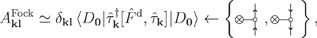(39)

or the diagonal part of the full Hamiltonian,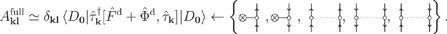(40)

The former is universally implemented in deterministic CC codes, and its effectiveness can be justified through perturbative arguments.59 The use of the latter has not, to the best of our knowledge, been attempted before.

The derivation here presented makes explicit connection with preconditioning, already discussed by some of us in connection with FCIQMC79 and unlinked CCMC.80 We will discuss how preconditioning is implemented for diagCCMC in Sec. IV C.

Finally, let us point out that Jarlebring et al. showed how a specific instance of a nonlinear eigenvalue problem is equivalent to a Rosenbrock-type discretization of an associated imaginary-time ODE.76 An adaptive time step integrator can be, thus, formulated based on convergence estimates similar to those presented in Ref. 76.

We wish to stochastically solve the linked CC equation (18b). Additionally, and at variance with the approach of Franklin et al., we wish to overcome the need for a corrected update step and the sampling of extraneous unlinked terms.43 Whereas the latter have been observed to cancel out on average, they impose limitations to what system sizes are approachable before the memory cost becomes prohibitive.

In the diagCCMC algorithm,47 we use the uncorrected update step in Eq. (30). Two novel insights allow us to achieve this goal:

• The CC wavefunction is stored in a compressed representation without invoking particles or walkers. It is comparatively easier to enforce constant unit intermediate normalization within a walker-less algorithm.

• The CC vector function appearing in the update step is an integral expressible as a terminating series expansion. Terms in this expansion can be evaluated stochastically.

The use of diagrammatic techniques automatically guarantees that only connected terms in the similarity-transformed Hamiltonian are included. The sampling will, thus, happen in “diagram space” and relies on the even selection algorithm of Scott et al.44

Previous algorithms to stochastically solve the linked CC equations modified the propagation in (30) to approach the correct solution. The need for such modifications can be attributed to the use of a variable intermediate normalization,

$|CCMC =N0⁡expT̃^N0|D0 ,$
(41)

where the additional normalization parameter N0 is constrained by the energy equation,

$N0⟨D0|H¯−ECC|D0⟩=0,$
(42)

and the unknown CC energy must be substituted by the shift S. At the beginning of the simulation, S = Eref and this causes the energy estimator to converge incorrectly prior to initialization of population control. However, upon closer inspection, the wavefunction ansatz in (41) is seen to be equivalent to the conventional CC ansatz with (a) overlap with the reference set to N0 and (b) all nonzero cluster amplitudes tk represented by values larger than $1N0$. The floating intermediate normalization can then be interpreted as an algorithmic choice to determine the granularity of representation during the calculation and achieve compression of the CC wavefunction. This choice is arbitrary and can be related back to the conventional CC ansatz. Assume then that the intermediate normalization is now a constant value ⟨D0|CCMC⟩ = N0, set as an input parameter to the calculation. At sufficiently small granularities, the calculation will spontaneously stabilize at a system-dependent population of walkers without the need for population control. The stochastic realization of the modified explicit Euler integration,

$t̃k[n+1]=t̃k[n]−δτN0ω̃k[n],$
(43)

would then

1. compress the cluster amplitudes to the selected granularity, by stochastically rounding those amplitudes for which $|t̃k|<1$ to $sgn(t̃k)×1$ or 0,

2. evaluate the CC vector function by taking a large enough number of samples such that diagrams in $ω̃k$ of magnitude 1 are, on average, selected once,

3. adjust the time step δτ as to avoid particle blooms, which are a large spawning event, which would destabilize the calculation dynamics.

We can, however, take one further step and cast away the walker interpretation entirely. The thresholding implied in the previous algorithmic sketch can be rigorously formulated without recourse to walkers. We introduce three strictly positive calculation parameters: the representation granularity Δ, the evaluation granularity γ, and the maximum diagram contribution ϵ. The algorithm then will

1. compress the cluster amplitudes to the chosen representation granularity, by stochastic rounding amplitudes for which |tk| < Δ to sgn(tk) × Δ or 0,

2. evaluate the CC vector function stochastically such that diagrams with magnitude γ are selected once on average,

3. adjust the time step δτ such that the maximum diagram contribution, $δτwdiagrampdiagram$, is of magnitude ϵ.

The walker and walker-less representations are entirely equivalent. The representation granularity is the inverse of the intermediate normalization constant $Δ=1N0$, the condition γ = Δ defines the even selection approach,44 and the ratio $ϵΔ$ is the maximum allowed size for a spawning event. The resultant approach to the imposition of sparsity bears some resemblance to recent fast randomized iteration approaches.81,82

Within this approach, the total walker population is the sum of rescaled cluster coefficient absolute magnitudes and the reference $1Δ+∑i|ti|Δ$. It is, thus, not needed to set the hard-to-predict total walker population as a calculation parameter: choosing to stochastically round all cluster coefficients below a certain value gives a more intuitively stable treatment between different calculations. The total walker population can vary dramatically with system size: evaluating and comparing computational cost and performance for systems of varying size can be a nontrivial challenge. Instead, we expect the walker-less picture to manifest the transferability property of cluster amplitudes:83 the magnitude of the amplitudes should be relatively unchanged with system size, especially when localized orbitals are used, allowing equivalent parameters for different calculations to be easily identified.

We have found γ = 10−3 to be the lowest evaluation granularity giving a calculation stable enough to extract statistics from. While smaller γ values achieve more stable calculations, with 10−4 providing a reasonable compromise between the computational cost and stability. We have also continued to use conventions from the particle representation for now by setting $Δγ=1$ and $ϵγ=3$.

The second essential insight enabling the diagCCMC algorithm is the stochastic evaluation of the CC vector function on the right-hand side of the uncorrected update step. At any given excitation level in the CC hierarchy, the BCH expansion of $H¯$ will truncate at the fourfold nested commutator: ωk is expressible as a sum of a finite enumerable number of terms. We choose to represent these terms as diagrams and generate such an expansion on the fly rather than enumerating the allowed diagrams beforehand. In each main Monte Carlo cycle in the algorithm, we perform the evaluation of the integral by attempting to select nafully specified diagrams from its expansion. The action of the similarity-transformed Hamiltonian on the reference determinant can be written compactly as

$H¯|D0 =wlτ^l|D0 ,$
(44)

where the amplitude $w$l = $w$Hmtm is a product of a one- or two-body integral from the Hamiltonian and a cluster of excitors. The multi-index l is fully specified, meaning that all hole and particle lines are explicitly labeled. The amplitude is determined by the contraction pattern randomly selected during diagram generation. Finally, since $⟨D0|τ^p†τ^q|D0⟩=δpq$, the selected diagram can contribute to one and only one cluster amplitude: the one whose multi-index corresponds to the external lines in $w$l. The rules for the deterministic enumeration of diagrams that were briefly detailed in Sec. II C are largely unmodified in our stochastic algorithm. Each step corresponds to an event occurring with an easily computed probability:

1. Sample the action of the wave operator: with probability psel, choose a term from the BCH expansion (20), that is, select a cluster of size N ≤ 4 and the excitation level of each constituent excitor. We use the even selection scheme of Scott et al.44 in a walker-less representation (see Sec. V A).

2. Sample the action of the Hamiltonian: with probability phver, choose one of the 13 interaction vertices in Table II. This step is not independent of the former, and we use importance sampling (see Sec. V A).

3. Sample the admissible contraction patterns: with probability pcont, choose a specific Kucharski–Bartlett sign sequence,60,61,63 see Table III, for example.

4. Sample the index set to label internal lines. Given the number of internal hole and particle lines in the selected contraction, the probability associated with this step pint is computed combinatorially.

5. Sample the index set to label external lines. As for the previous step, the probability pext is also computed combinatorially.

TABLE III.

Generation of diagrams stemming from the $(ΦT12T3)c$ term and contributing to the triples equations. The Kucharski–Bartlett sign sequences for excitors and interaction vertices, resulting contraction patterns, and resulting diagrams are shown.

ExcitorsInteractionContractionDiagram
+ −| + −| + + + −−− + + −− + −| + |−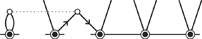+ −|−| +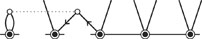+ |−−| +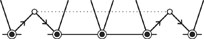−| + + |−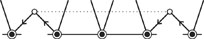+ |−| + −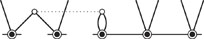ExcitorsInteractionContractionDiagram
+ −| + −| + + + −−− + + −− + −| + |−+ −|−| ++ |−−| +−| + + |−+ |−| + −With this process, we are able to obtain a given diagram with probability pdiagram = pselectphverpcontpintpext, and in each Monte Carlo step, the diagram is sampled pdiagram × na times.

We need further minor modifications to the deterministic enumeration of diagrams to ensure that the CC vector function is evaluated correctly. Our algorithm singles out specific diagrams, where all lines, internal and external, are explicitly labeled. This procedure identifies a single cluster amplitude tk to which the selected diagram will contribute without having to sum over internal lines. Permutational symmetries will, thus, have to be handled differently such that our probability distributions are properly normalized. Sums of the form $12∑i,j$ have to be replaced with $∑i>j+12δij$ to ensure that there is only a single way to select diagrams related by the following:

• the antipermutation of indices stemming from antisymmetrized interaction vertices,

• the antipermutation of hole or particle indices stemming from excitor vertices, and

• the commutation of excitors.

For the first two cases, terms with i = j would vanish when summing over equivalent indices. In the last case, the diagonal case i = j indicates additional symmetries of the resulting diagram. In our stochastic diagram enumeration, each pair of equivalent internal or external lines will not require a $12$ factor. Moreover, upon selection, a well-defined ordering of excitors is established, which removes the need for $12$ factors in diagrams where excitors of the same rank appear. These modifications to the deterministic evaluation rules ensure a unique selection of a contraction pattern.60,61,63 The action of permutation operators for inequivalent external lines is subsumed into the permutation of hole and particle indices needed to store the result of the sampling in antisymmetrized ordering, which provides the appropriate parity factor (−1)σ. With these considerations, a contribution to tk is computed as

$xdiagram=wdiagrampdiagram=(−1)σwTwHpselectphverpcontpintindspextinds,$
(45)

and the sampling algorithm is designed such that pdiagram = |$w$diagram|. Ultimately, our aim is to achieve importance sampling between contributions (see Sec. V C).

While the imaginary-time propagation discussed in Sec. III will generally be used within our work, we could also make use of arbitrary preconditioners. Apart from the identity, we implemented two additional options: the diagonal of the Fock operator (diagrams 1 and 2 in Table II) and the diagonal of the full Hamiltonian (diagrams 1, 2, 4, 5, and 6 in Table II). The connected portions of these vertices do not modify excitors when applied. These preconditioners are the iteration-independent approximations to the Jacobian discussed in Sec. III B and strike a balance between the computational complexity and improvement of convergence. The diagonal Fock preconditioner is ubiquitously implemented in deterministic CC approaches.59 Since all relevant quantities are precomputed, the values of either preconditioner can be evaluated with a cost independent of the system size, unlike the implementation of the similar approach within FCIQMC and CCMC.79,80

The portion of the Hamiltonian used for preconditioning can then be applied via a straightforward rescaling of the original cluster amplitudes by a factor of 1 − δτ. The remainder of the Hamiltonian is applied explicitly, as in the imaginary-time propagation, before rescaling by the preconditioner.

We will not investigate the benefits of preconditioning here but wanted to observe that the diagrammatic formalism lends itself to a straightforward implementation of a range of preconditioners without introducing additional computational costs scaling with system size. This results from the use of the connected portions of all preconditioners, unlike previous stochastic approaches.79,80

The even selection algorithm was proposed by Scott and Thom44 to improve the sampling of the action of the CC wave operator on the reference determinant. Even selection was specifically designed to alleviate calculation instabilities due to the occurrence of large particle blooms. Sampling proceeds via the selection of clusters containing a specific number of excitors of each rank, termed a combination, separately. In this section, we summarize the adaptation of even selection in a walker-less context. We then illustrate the need for the importance sampling of $(H⁡expT)c$ and describe the strategy implemented in diagCCMC.

The original even selection algorithm defined the probability of selecting a particular set of excitors e from the combination c of size s as

$pselect(e)=psize(s)pcombo(c|s)pexcitors(e|c,s),$
(46)

a series of conditional probabilities. We re-express the selection probability as follows:

$pselect(e)=pcombo(c)pexcitors(e|c),$
(47)

to simplify considerations to follow. We also assume the unit intermediate normalization.

We adopt the same notation used in Ref. 44 and denote the number of excitors of rank j within combination c as ηcj. Lj is the sum of absolute magnitudes of cluster amplitudes at rank j, that is, the 1-norm of Tj.

In keeping with the original approach, ηcj denotes the number of excitors of rank j contained within combination c, Lj is the sum of cluster coefficient absolute magnitudes at rank j, and na is the number of sampling attempts to be made for that iteration.

In the walker-less representation, the amplitude of a given cluster is the product of cluster coefficients $w$e = ∏ptp. The evaluation granularity is, by definition, equal to the absolute magnitude of the MC weight,

$|xe|=|we|napselect(e)=γ.$
(48)

Even selection for all clusters requires that evaluation and representation granularities be the same: γ = Δ. As we also require $|we|pselect(e)$ to be an excitor-independent constant, we obtain

$pexcitors(e|c)=∏j=1lηcj!∏p∈ejηcj|tp|Lj,$
(49)
$pcombo(c)=1W∏j=1lLjηcjηcj!$
(50)

with normalization constant

$W=∑cncombo∏j=1lLjηcjηcj!,$

where ncombo is the total number of combinations. The number of random samples to take within a calculation is, thus, obtained from the evaluation granularity given as input,

$na=Wγ.$
(51)

Each pairing of excitor combinations with Hamiltonian vertices can result in a different number of admissible contractions and, thus, fully indexed diagrams. It is non-trivial to ensure that |xdiagram| in (45) is in any sense comparable between the different pairings. The selection of a Hamiltonian interaction vertex is not independent of the selection of excitor combination: phver will be a probability conditional on pselect. This enables the use of truncated excitation generation, that is, the a priori exclusion of Hamiltonian–excitor pairings that will not be able to contribute to any stored amplitude.84 Furthermore, one could easily exclude any class of diagrams that we wish to evaluate with a different algorithm.

Clusters from different combinations can contribute to a set of allowed diagrams, whose number can undergo large variations, especially with varying system size. In a CC calculation to any order, let us consider two limiting cases in the sampling to clarify this statement. Assume that we selected a cluster from the combination $T14$ with no repeated excitors. The only fully connected diagrams stemming from such a cluster are of the form

as such

1. only one Hamiltonian interaction vertex is admissible:

with probability phver = 1.0.

2. selecting a contraction pattern boils down to the choice of which two excitors from the four to be connected to the interaction vertex via hole-type lines. There are $42=6$ possible ways of doing so, which gives $pcont=16$.

3. being single excitations, each excitor has one particle and one hole line. Once the contraction pattern is set, there is only one choice to make per hole line in the diagram and each will be made with probability pint = 1.0.

4. as all external indices are fully determined by the selected cluster and contraction, there is only a single possible choice of external indices, so pext = 1.0.

Each cluster from a $T14$ combination can contribute to six valid diagrams, independent of the system size and truncation level. If all diagrams are selected without weighting, $pdiagram=16pselect$.

Now assume, instead, that we are sampling the action of a bare Hamiltonian vertex, that is, a cluster of size 0. There are only two admissible choices in such a case,

There is no contraction to decide upon and, hence, no internal indices to decide upon: pcont = 1.0 = pint. However, the amount of such terms to sample varies with system size. For an N-electron system with V virtual orbitals, there are O(N) possible external hole and O(V) possible external particle indices, respectively. For a one-body interaction vertex, there are O(NV) admissible labelings of the diagram, while for the two-body case, there are O(N2V2) such labelings.

These examples show how sampling different classes of diagrams will require a varying number of attempts na in each MC step. The original even selection prescription will need to be modified to accommodate this, or else calculations will rapidly become untenably expensive.

To compensate for the difference in diagram generation between different combinations, we will now modify our sampling to include combination-dependent constants αc,

$0.0<αc≤1.0, max(αc)=1.0,$
(54)

such that

$pcombo(c)=αcW∏j=1lLjηcjηcj!, W=∑cncomboαc∏j=1lLjηcjηcj!.$
(55)

Each combination will be evaluated to a different granularity γc, defined as in (48),

$γc=|we|napselect(e)e∈c=Wnaαc=γαc,$
(56)

where we used the value of na given in (51).

With this modification, we now require an approximately constant contribution to the integrals $D0|τ^k†H¯|D0$. From Sec. IV B, we know the diagram amplitude,

$|xdiagram|=|we|napselect(e)|wH|phverpcontpintpext,$
(57)

must then be a constant Ξ. This constant is a product of interaction vertex-specific and diagram-specific factors,

$Ξ=γcζciBci,$
(58)

where ζci is the probability of choosing the ith Hamiltonian interaction vertex (Table II) when sampling excitor combination c and

$Bci=|wH|pcontpintpext.$
(59)

The ζci probabilities are normalized, ∑iζci = 1.

Unfortunately, having all contributions be of constant magnitude is not a tenable aim. We can, instead, aim to have either the average or maximum contribution from the sampling of each combination with each admissible interaction vertex being a constant value. We, thus, require

$Ξ=γcζciBci⋆=γαcζciBci⋆,$
(60)

where $Bci⋆$ can be either the maximum or the average value for the diagram-specific term. Considering the maximum contribution, we then obtain

$γci=Bcimax∑jBcjmax,$
(61a)
$χc=Bcimaxζci,$
(61b)
$αc=χcmax(χc),$
(61c)
$Ξ=γmax(χc),$
(61d)

and similarly, for the average contribution involving $Bciave$. Utilizing these expressions requires on-the-fly accumulation of the values $Bci⋆$ during a calculation. Ξ will be the value of the maximum (average) contribution for all diagrams using admissible excitor combinations and interaction vertices before scaling by the time step δτ. The latter can be set according to

$δτ=ϵΞ=ϵγmax(χc),$
(62)

where the parameter ϵ was introduced in Sec. IV A and χc is calculated using $Bcimax$. Using $Bciave$ would set the time step such that the average contribution magnitude was ϵ.

The accumulated values for the diagram-specific terms $Bci⋆$ guarantee that this procedure uses information from all valid diagrams ever generated, whether spawning attempts were successful or not. As such, it can converge to a stable importance sampling of the wavefunction with minimal user input. To avoid certain classes of diagrams being entirely neglected as a result of a single negligible diagram, the values γci must be fixed until sufficient information has been collected. This is achieved by requiring 100 random diagrams of each type be selected during the calculation before starting the importance sampling procedure.

The application of truncated excitation generation, as introduced in Sec. V B, follows naturally in this algorithm and provides considerable computational benefits.

Most notably, while the time step is still expected to decrease as $ON−4$ due to the sampling of the bare Hamiltonian, HN, the cost of sampling larger clusters will rapidly fall. This is due to the number of possible diagrams for higher excitation level clusters having a scaling lower than $ON4$, and so αc correspondingly falling with system size to compensate.

As an example, selected clusters of excitation level l + 2 only have $O1$ possible diagrams due to the restriction to connected diagrams contributing to the excitation level l or below. The first example discussed in Sec. V B corresponds to this case in CCSD. To compensate for this, αc will fall as $ON−4$ for this combination as fewer samples are required. This gives the overall scaling of sampling these higher terms as $ON2l+4$ for this case. For clusters of lower excitation level, sampling the cluster expansion will have reduced computational expense, which will, however, be offset by a corresponding increase in the number of connected diagrams these clusters can contribute to. This differs from prior unlinked approaches, where all Hamiltonian vertices are sampled for every cluster, so every cluster effectively contributes to $ON4$ (possibly disconnected) diagrams. This results in an additional factor of $ON4$ in the computational scaling of unlinked approaches compared to diagCCMC.

As such, the overall cost of a diagCCMC calculation will scale as $ON2l+4$ for a fixed errorbar per electron in the absence of any simplifying properties of the cluster amplitudes. This gives an asymptotic scaling of $ON8$ for CCSD with a fixed errorbar per electron. The asymptotic scaling matches that of deterministic unfactorized CC theory, unlike prior stochastic CC approaches.44 If a fixed errorbar were instead required, this would lead to a scaling of $ON9$ for CCSD and $ON2l+5$ in general.

In previous work,47 we demonstrated that for systems of noninteracting replicas, the memory cost for diagCCMC is proportional to the number of replicas, regardless of the truncation level of the theory, and that this extends to interacting systems provided cluster amplitudes decay sufficiently rapidly with distance. Here, we extend this consideration to show that the computational effort will asymptotically scale as at most$ON4$ in the presence of locality, regardless of the truncation level, for a fixed errorbar per electron.

Our only requirement is that cluster amplitudes be homogeneous: the absolute magnitude of all $T^m$ is proportional to some measure of system size, N, as is expected to be the case when locality is present, for instance, in insulators over reasonable length scales. This means that we can sample the contribution of a cluster of n excitors to a fixed granularity using only $ONn$ random samples. The linked diagram theorem then restricts us to clusters containing at most 4 excitors and ensures that the number of “free,” external coupling indices on the Hamiltonian coupling vertex that must be sampled is at most 4 − n. Sampling each external index will require $ON$ additional samples of that diagram, so the maximal scaling to sample a cluster of size n(≤4) is $ONnON4−n=ON4$. This scaling will be reflected in the number of attempts per unit of imaginary time, naδτ−1, and is independent of the chosen truncation level in the CC hierarchy. In the case of noninteracting replicas, the computational effort per replica, $naδτ−1nreplicas−1$, is expected to scale as $ON3$, again independent of the truncation level.

It is important to note here a benefit of the stochastic approach that the sparsity and structure within the cluster amplitudes are exploited automatically, but only if they are present. In the absence of such a structure, the result will still be equivalent to a conventional CC calculation. This is different from local deterministic approaches, which by necessity neglect nonlocal contributions according to some categorization. If locality is not present to the appropriate degree, such approaches will obtain a different answer from a conventional CC calculation on the same system. While this may seem technical, being able to exploit locality while still estimating the exact CC energy is a considerable benefit.

Our diagCCMC algorithm is implemented in a standalone package. The package is written in the Python programming language, which allows fast prototyping and experimentation.

A sparse stochastic array is the basic data structure. This is used to store the compressed representation of cluster operators Tm of any rank. We use a Python dictionary, an associative key-value array implemented as a hash table.85 The excitation indices are the keys,

$ta1a2…aki1i2…ik⟼((i1,i2,…,ik),(a1,a2,…,ak)):t,$
(63)

with cluster amplitudes stored as floating-point numbers. The key is arranged as a 2-tuple of k-tuples: each k-tuple representing the hole and particle indices, respectively. This sparse stochastic array is designed to (a) be exchange symmetry-aware, (b) perform stochastic rounding to a preset threshold, Δ, and (c) enable the importance sampling of its elements. The keys in the dictionary are sorted in ascending order, both in the hole and particle tuples, which ensures no storage redundancy. Upon insertion in the data structure, the supplied index is first sorted in ascending order, while the supplied value is multiplied by the corresponding parity phase factor, (−1)σ. The new value is inserted after stochastic rounding,

$|t|<Δ⇒t=(−1)σsgn(t)Δ if UniformRandom[0,1]<|t|Δ0 if UniformRandom[0,1]>|t|Δ,$
(64)

and finally, the 1 norm of the Tm cluster operator is updated accumulating the new value: $∥$Tm$∥$1 ← abs(value). Similarly, upon lookup, the supplied index is first sorted and then looked up into the dictionary. If present, the returned value accounts for the parity phase factor. Iteration and various vector-like operations can be implemented on top of this storage object. Compressed sparse matrix formats could replace the hash table. However, the algorithm is not GEMM-driven,62 and compressed sparse representation would be suboptimal for importance sampling. Insertion and retrieval are the essential operations in our algorithm, and they can be performed on a hash table with $O1$ complexity in the average case. Furthermore, data needed for importance sampling can be accumulated upon insertion into the hash table, eliminating the need for complete traversals of the data structure.

The importance sampling of the data in the sparse stochastic array requires building the corresponding sampling distribution, either using a cumulative magnitude array or the alias method86,87 with the Vose sampler.88 If n is the number of elements in the discrete set to sample, the alias method constructs the distribution in $On$, while sampling is achieved in $O1$.

The cluster operator T is a collection of sparse stochastic arrays, indexed on the rank of its constituent excitations. Various vector-like algebraic operations can be implemented for this data structure, e.g., the calculation of the 1 and 2 norms of T, in the form of reductions over the sparse stochastic arrays of the component operators.

Finally, we handle the importance sampling described in Sec. V C in a separate data structure: the sampling store. This data structure computes the probabilities for the selection of excitor combinations, pcombo,44 and for the selection of a combination–Hamiltonian vertex pairing in diagram generation, phver, the latter being conditional on the former. The sampling store is also responsible for accumulating data needed to update the sampling distributions, which is further used to determine the time step for the next iteration.

We show a high-level overview of diagCCMC in Algorithm 1. A diagCCMC calculation requires as input the following:

• Molecular integrals in an orthogonal molecular orbital (MO) basis. These are expected in FCIDUMP format.89

• A truncation level for the cluster operator.

• The number of steps, NQMC, to perform.

• The stochastic granularity, Δ, which defaults to 10−4 in our implementation.

• The time step, δτ, which defaults to 0.01 in our implementation.

• The preconditioner, which defaults to the identity in our implementation.

ALGORITHM 1

High-level overview of the diagCCMC algorithm. The main Monte Carlo loop in the calculation is initialized given a reference single determinant and its Hamiltonian matrix elements in FCIDUMP format.89 The MP1 amplitudes are used as the initial t guess.

 1: procedure diagCCMC(H, t, NQMC) 2: Initialize sparse stochastic storage for T 3: Bootstrap importance sampling of T 4: for n < NQMC do 5: Update sampling distribution for T[n] ⊳ see Sec. V A 6: Stochastic propagation ⊳ see Algorithm 2 7: Compute the energy deterministically: $ΔECC=∑aitaifia+14∑ijabtabijg¯ijab+12∑ijabtaitbjg¯ijab$ 8: Update T[n+1] ← ω[n] (“annihilation”) ⊳ see Sec. IV C 9: end for 10: end procedure
 1: procedure diagCCMC(H, t, NQMC) 2: Initialize sparse stochastic storage for T 3: Bootstrap importance sampling of T 4: for n < NQMC do 5: Update sampling distribution for T[n] ⊳ see Sec. V A 6: Stochastic propagation ⊳ see Algorithm 2 7: Compute the energy deterministically: $ΔECC=∑aitaifia+14∑ijabtabijg¯ijab+12∑ijabtaitbjg¯ijab$ 8: Update T[n+1] ← ω[n] (“annihilation”) ⊳ see Sec. IV C 9: end for 10: end procedure

The CC wavefunction is initialized using the MP1 amplitudes, easily computed from the provided MO basis integrals. Both the representation of the CC wavefunction at the current time step and its update (the residual) are represented as stochastic sparse arrays, but only the former will be used for sampling purposes. We initialize importance sampling with a short trial run to sample all possible pairings of excitor combinations and Hamiltonian vertices.

Each MC cycle starts by updating the sampling distribution for the cluster operator, and we leverage information about selection probabilities for each Hamiltonian vertex with each excitor combination, accumulated from diagram generation attempts in the previous cycle, to define an importance sampling weight for each combination of excitors.

The stochastic propagation step performs na attempts at sampling the CC residual, ω[n], constructing diagrams on the fly and is schematically described in Algorithm 2. Our current implementation features a process-based parallelization of this step. Given p helper processes, each available helper performs $⌈nap⌉$ attempts and stores their results in a queue.90 These are aggregated by the main process, which also takes care of cleaning up the queue before entering the next QMC step in the simulation. For each attempt, we first obtain a random cluster and accumulate its relevant contributions to estimators, e.g., the energy. Given the cluster, we sample its diagonal and off-diagonal actions, which we term “death” and “spawn” attempts, respectively, in analogy with existing Fock-space QMC terminology. The “death” step consists of the exact evaluation of all components of the Hamiltonian, which results in contributions to the same excitor, as was originally sampled, provided these have not been incorporated into the preconditioner, as discussed in Sec. IV C. This consists of contributions from vertices 1, 2, 4, 5, and 6 in Table II. We may sample exclusion-principle violating (EPV) diagrams64 within “death.” These would cancel out exactly in a deterministic evaluation and are, thus, not stored into the sparse representation of the cluster operator.

ALGORITHM 2

Stochastic propagation.

 1: procedure Diagrams(H, T[n], na) 2: for i < na do 3: Obtain random cluster ti…tl from T[n] 4: Accumulate contribution of selected cluster to estimators 5: Sample diagonal action of cluster (“death”) 6: if “death” diagram is not EPV then 7: Apply preconditioning ⊳ see Sec. IV C 8: Store in residual object after stochastic rounding 9: end if 10: Sample off-diagonal action of cluster (“spawn”) ⊳ see Algorithm 3 11: if “spawn” successful then 12: Evaluate diagram 13: Apply preconditioning ⊳ see Sec. IV C 14: Store in residual object after stochastic rounding 15: end if 16: Accumulate “spawn” statistics for importance sampling 17: end for 18: end procedure
 1: procedure Diagrams(H, T[n], na) 2: for i < na do 3: Obtain random cluster ti…tl from T[n] 4: Accumulate contribution of selected cluster to estimators 5: Sample diagonal action of cluster (“death”) 6: if “death” diagram is not EPV then 7: Apply preconditioning ⊳ see Sec. IV C 8: Store in residual object after stochastic rounding 9: end if 10: Sample off-diagonal action of cluster (“spawn”) ⊳ see Algorithm 3 11: if “spawn” successful then 12: Evaluate diagram 13: Apply preconditioning ⊳ see Sec. IV C 14: Store in residual object after stochastic rounding 15: end if 16: Accumulate “spawn” statistics for importance sampling 17: end for 18: end procedure

During the “spawn” attempts, on-the-fly diagram generation will occur, as described in Algorithm 3. Note that this algorithm is short-circuiting: an unsuccessful random selection at any step will return an empty diagram and result in the accumulation of a failed attempt.

ALGORITHM 3

Diagram generation.

 1: procedure diagram generation(ti…tl, sampling distribution, |D0⟩) 2: Select Hamiltonian vertex 3: Select contraction pattern 4: Select internal indices 5: Select external indices ⊳ spin-conservation constraints are enforced 6: end procedure
 1: procedure diagram generation(ti…tl, sampling distribution, |D0⟩) 2: Select Hamiltonian vertex 3: Select contraction pattern 4: Select internal indices 5: Select external indices ⊳ spin-conservation constraints are enforced 6: end procedure

Once a diagram has been selected, its evaluation is done deterministically by applying the algebraic interpretation rules with modifications described in Sec. IV B. The energy is also evaluated deterministically, but note that a stochastic estimator can also be built during “death” and “spawning” steps. Finally, the cluster operator is updated before moving on to the next MC cycle, taking into account the preconditioning of the residual (see Sec. IV C).

To demonstrate the retention of the favorable properties of our approach when applied to higher excitation levels in systems of multireference character, we consider calculations including up to quadruple excitations upon H4, in a square of side length 1.5 Å. At this geometry, two restricted Hartree–Fock (RHF) solutions are degenerate.91 Any single configuration provides only a poor representation of the system, while coupled cluster with up to quadruple substitutions (CCSDTQ) is equivalent to FCI. Each truncation level has a clearly identifiable difference in energy, which can be resolved despite stochastic error. We also consider noninteracting replicas of this system such that the wavefunction will become a product.

This system, while small, is by no means trivial for a projection-based approach. Its multireference nature and small gap between the ground and excited states necessitates projection through over 50 units of imaginary time to converge to the ground state. The imaginary-time propagation was not preconditioned. While preconditioning can afford taking larger time steps,79 we observed that it can lead to an unstable propagation in this particularly challenging case.

The resultant energies are shown in Table IV, demonstrating the size-extensivity of the energies, within stochastic errorbars, for multiple noninteracting replicas.

TABLE IV.

Correlation energies (Eh) of H4 and noninteracting replicas systems at different CC truncation levels in a 6-31G basis. Molecular integrals were generated in FCIDUMP format89 with the psi4 program package.92 Deterministic energies calculated using MRCC93 for a single replica are −0.167 46 Eh, −0.169 98 Eh, and −0.162 23 Eh for CCSD, CCSDT, and CCSDTQ, respectively.

nreplicasCCSDCCSDTCCSDTQ
−0.1678(2) −0.1701(2) −0.1624(2)
−0.3353(3) −0.3398(3) −0.3242(8)
−0.5022(8) −0.5129(4) a
−0.6688(7) a a
nreplicasCCSDCCSDTCCSDTQ
−0.1678(2) −0.1701(2) −0.1624(2)
−0.3353(3) −0.3398(3) −0.3242(8)
−0.5022(8) −0.5129(4) a
−0.6688(7) a a
a

Values not computed due to computational constraints.

The memory cost per replica, as measured by the nstates metric, is shown in Fig. 1. The $ON$ asymptotic scaling for noninteracting systems was already observed in Ref. 47 for noninteracting Be replica systems and is confirmed here also for the H4 systems. As discussed in Sec. V D, this is an intrinsic property of the diagCCMC algorithm, and our results confirm that it is preserved even in cases where the description of the electronic structure is challenging.

FIG. 1.

nstates per replica for noninteracting replicas of H4 in a square geometry of side length 1.5 Å in a 6-31G basis. The nstates metric is a measure of the memory cost of the calculation. Molecular integrals were generated in FCIDUMP format89 with the psi4 program package.92 Missing points were not computed due to computational constraints.

FIG. 1.

nstates per replica for noninteracting replicas of H4 in a square geometry of side length 1.5 Å in a 6-31G basis. The nstates metric is a measure of the memory cost of the calculation. Molecular integrals were generated in FCIDUMP format89 with the psi4 program package.92 Missing points were not computed due to computational constraints.

Close modal

The naδτ−1 metric measures, instead, the computational requirements per replica and is shown in Fig. 2. From the discussion in Sec. V D, this is expected to scale cubically with nreplicas.

FIG. 2.

Number of stochastic samples performed (na) per unit imaginary time per replica for noninteracting replicas of H4 in a square geometry of side length 1.5 Å in a 6-31G basis. This metric is proportional to the CPU cost required to obtain a fixed errorbar per electron. Molecular integrals were generated in FCIDUMP format89 with the psi4 program package.92 Missing points were not computed due to computational constraints.

FIG. 2.

Number of stochastic samples performed (na) per unit imaginary time per replica for noninteracting replicas of H4 in a square geometry of side length 1.5 Å in a 6-31G basis. This metric is proportional to the CPU cost required to obtain a fixed errorbar per electron. Molecular integrals were generated in FCIDUMP format89 with the psi4 program package.92 Missing points were not computed due to computational constraints.

Close modal

The results of a log-linear regression analysis of the observed naδτ−1 against nreplicas are reported in Table V. We also include the same analysis on similar data obtained for Be in Ref. 47. All observed scaling exponents are below the expected maximum scaling of $ON3$. This is not a surprising result: we are not in the asymptotic large-system limit, and the highest-scaling contributions will not necessarily dominate the computational cost.

TABLE V.

Scaling exponents and prefactors for the computational scaling of diagCCMC calculations with respect to the number of replicas in systems on noninteracting replicas at various CC truncation levels. The computational scaling is estimated with the naδτ−1 metric discussed in the main text. For a dependency $naδτ−1=cnreplicasα$, we fit the linearized model ln(naδτ−1) = α ln nreplicas + ln c. Scaling parameters for noninteracting H4 replicas and noninteracting Be replicas are presented. The data for the latter are from Ref. 47 and can be found at https://doi.org/10.17863/CAM.34952. We performed all model fitting using the SciPy package.94

SystemTruncationln cα
H4 CCSD 12.73 2.39 ± 0.06
CCSDT 13.98 2.53 ± 0.06
CCSDTQ 14.70 2.54 ± 0.00
Be CCSD 12.76 2.75 ± 0.04
CCSDT 13.72 2.85 ± 0.03
CCSDTQ 14.00 2.88 ± 0.02
SystemTruncationln cα
H4 CCSD 12.73 2.39 ± 0.06
CCSDT 13.98 2.53 ± 0.06
CCSDTQ 14.70 2.54 ± 0.00
Be CCSD 12.76 2.75 ± 0.04
CCSDT 13.72 2.85 ± 0.03
CCSDTQ 14.00 2.88 ± 0.02

Finally, we also present correlation energies for the symmetric double dissociation of water in a 6-31G basis (see Table VI). In this system, different correlation regimes are in effect along the potential energy surface, and it is, thus, one of the standard benchmarks for correlated methods.95 diagCCMC manages to reproduce values obtained with deterministic approaches at a range of truncation levels along the binding curve. As is the case for the H4 calculations presented earlier, the multireference nature of this problem at certain stretched geometries did not allow some of these more challenging calculations to complete. The diagCCMC algorithm is a projection method; despite its intrinsic computational benefits, it still struggles when applied to problems with an ill-defined single reference determinant and/or characterized by a small gap.

TABLE VI.

Correlation energies (Eh) of the double dissociation of H2O at different CC truncation levels in a 6-31G basis. Molecular integrals were generated in FCIDUMP format89 with the psi4 program package.92 Geometries were taken from Ref. 95 with Re = 1.843 45 a0.

RO–H/ReCCSDCCSDTCCSDTQ
1.0 −0.136 58(5) −0.137 94(8) −0.1380(2)
1.5 −0.194 3(1) −0.199 7(4) −0.2008(5)
2.0 −0.290 6(2) −0.303 2(3) a
3.0 −0.531 5(5)b −0.550 3(6) a
RO–H/ReCCSDCCSDTCCSDTQ
1.0 −0.136 58(5) −0.137 94(8) −0.1380(2)
1.5 −0.194 3(1) −0.199 7(4) −0.2008(5)
2.0 −0.290 6(2) −0.303 2(3) a
3.0 −0.531 5(5)b −0.550 3(6) a
a

Values not computed due to computational constraints.

b

The calculation initially converges to the “canonical” CCSD solution, before decaying to a different solution with ΔECCSD = −0.5192(8) Eh after 80 a.u. of imaginary time. This property of the imaginary-time propagation has been noted before.47

We have discussed in detail our new approach for a stochastic solution of the linked coupled cluster equations and demonstrated the resulting reduction in computational and memory costs with system size in the presence of locality. The diagrammatic coupled cluster Monte Carlo algorithm uses the rigorously order-by-order and term-by-term size-extensive linked formulation of coupled cluster theory and ensures the efficient sampling of it by on-the-fly construction of coupled cluster diagrams. The algorithm is made possible by two novel insights: (a) the stochastic compression of multidimensional vectors can be achieved without invoking walkers and populations and (b) the CC vector function is an integral, expressible as a finite sum of diagrams, that can be computed by Monte Carlo sampling. Both insights lead to an algorithm that clarifies how randomness and sampling can be effectively leveraged to solve the high-dimensional nonlinear CC problem with a lower memory footprint and a more favorable operation count. The use of the well-known diagrammatic theoretical framework clarifies few points of the CCMC methodology, such as the relation of imaginary-time evolution to iterative solvers and the use of preconditioning.79,80 The representation and evaluation granularity parameters characterize the diagrammatic approach on a spectrum between fully deterministic and fully stochastic; the same theoretical framework can accommodate different numerical approaches. This paves the way for further cross-adaptation of deterministic and Monte Carlo techniques. The approach we have presented uses a naïve enumeration of diagrams: the residual is evaluated in its unfactorized nonlinear form,60,61 rather than the more computationally advantageous factorized quasilinear form.66,96 As such, it exhibits a high operation count, theoretically higher than that of its deterministic counterpart for a given excitation level if all cluster amplitudes are homogeneous. Deterministically, one would rather implement a quasilinear factorization with an optimal space–time trade-off.66 We are currently investigating this approach. The use of the diagrammatic expansion also paves the way for a rigorous derivation of a semistochastic CC method, where important residual components are resolved on the fly to machine accuracy, with the remainder only resolved to a preset stochastic representation granularity.

C.J.C.S. is grateful to Dr. George Booth for his current role as Postdoctoral Research Associate under Grant Agreement No. 759063 of the European Union’s Horizon 2020 research and innovation program. R.D.R. acknowledges partial support by the Research Council of Norway through its Centres of Excellence scheme, Project No. 262695, and through its Mobility Grant scheme, project number 261873. T.D.C. was supported by Grant No.CHE-1900420 from the U.S. National Science Foundation. A.J.W.T. is grateful to the Royal Society for a University Research Fellowship under Grant Nos. UF110161 and UF160398. R.D.R. thanks Simen Kvaal (University of Oslo) for pointing out Ref. 76.

We used the goldstone LATEX package to draw the coupled cluster diagrams. The package is available on https://github.com/avcopan/styfiles

All data and the code used for generation and analysis are freely available at doi.org/10.5281/zenodo.3997299.

Consider an operator $Ô$ dependent on a parameter λ, its derivative with respect to λ can be obtained as97

$∂∂λexpÔ(λ)λ=λ′=limδ→01δexpÔ(λ′+δ)−expÔ(λ′)≃limδ→01δexpÔ(λ′)+Ô̇(λ′)δ −expÔ(λ′)$
(A1)

since to first order in δ one has $Ô(λ′+δ)=Ô(λ′)+Ô̇(λ′)δ$. The differential $dexpÔ(λ′)=expÔ(λ′)+Ô̇(λ′)δ−expÔ(λ′)$ can be recast as a BCH series. Let us drop the λ′ dependence and rewrite the differential as

$dexpÔ=expÔexp−ÔexpÔ+Ô̇δ−1=expÔexp−Ôzexp[Ô+Ô̇δ]z01=expÔ∫01 dz d dzexp−Ôz −exp(Ô+Ô̇δ)z.$
(A2)

We can calculate the z-derivative as

$d dzexp−Ôzexp(Ô+Ô̇δ)z =exp−Ôz{Ô̇δ}exp(Ô+Ô̇δ)z ≃δ⁡exp−Ôz{Ô̇}expÔz,$
(A3)

where in the last step we dropped O(δ2) terms. We then expand the last term in a BCH series,

$exp−Ôz{Ô̇}expÔz=∑n≥01n!(Ô̇Ôz̲n), (Ô̇Ôz̲)=def[Ô̇,Ôz].$
(A4)

We exchange summation and integration orders and perform the z-integration to obtain

$dexpÔ=δ⁡expÔ∑n≥01(n+1)!(Ô̇Ô̲n)$
(A5)

such that the λ-derivative is

$∂∂λexpÔ=expÔ∑n≥01(n+1)!(Ô̇Ô̲n).$
(A6)
1.
W. M. C.
Foulkes
,
L.
Mitas
,
R. J.
Needs
, and
G.
Rajagopal
,
Rev. Mod. Phys.
73
,
33
(
2001
).
2.
J.
Kolorenč
and
L.
Mitas
,
Rep. Prog. Phys.
74
,
026502
(
2011
).
3.
C. J.
Umrigar
,
J. Chem. Phys.
143
,
164105
(
2015
).
4.
B. M.
Austin
,
D. Y.
Zubarev
, and
W. A.
Lester
,
Chem. Rev.
112
,
263
(
2012
).
5.
R. J.
Hunt
,
M.
Szyniszewski
,
G. I.
Prayogo
,
R.
Maezono
, and
N. D.
Drummond
,
Phys. Rev. B
98
,
075122
(
2018
).
6.
P.
Pulay
,
Chem. Phys. Lett.
100
,
151
(
1983
).
7.
S.
Saebo
and
P.
Pulay
,
Annu. Rev. Phys. Chem.
44
,
213
(
1993
).
8.
C.
Hampel
and
H. J.
Werner
,
J. Chem. Phys.
104
,
6286
(
1996
).
9.
M.
Schütz
and
H.-J.
Werner
,
J. Chem. Phys.
114
,
661
(
2001
).
10.
F.
Neese
,
F.
Wennmohs
, and
A.
Hansen
,
J. Chem. Phys.
130
,
114108
(
2009
).
11.
M.
Ziółkowski
,
B.
Jansík
,
T.
Kjaergaard
, and
P.
Jørgensen
,
J. Chem. Phys.
133
,
014107
(
2010
).
12.
K.
Kristensen
,
M.
Ziółkowski
,
B.
Jansík
,
T.
Kjærgaard
, and
P.
Jørgensen
,
J. Chem. Theory Comput.
7
,
1677
(
2011
).
13.
I.-M.
Høyvik
,
K.
Kristensen
,
B.
Jansik
, and
P.
Jørgensen
,
J. Chem. Phys.
136
,
014105
(
2012
).
14.
C.
Riplinger
and
F.
Neese
,
J. Chem. Phys.
138
,
034106
(
2013
).
15.
C.
Riplinger
,
B.
Sandhoefer
,
A.
Hansen
, and
F.
Neese
,
J. Chem. Phys.
139
,
134101
(
2013
).
16.
J. J.
Eriksen
,
P.
Baudin
,
P.
Ettenhuber
,
K.
Kristensen
,
T.
Kjærgaard
, and
P.
Jørgensen
,
J. Chem. Theory Comput.
11
,
2984
(
2015
).
17.
D. G.
Liakos
,
M.
Sparta
,
M. K.
Kesharwani
,
J. M. L.
Martin
, and
F.
Neese
,
J. Chem. Theory Comput.
11
,
1525
(
2015
).
18.
C.
Riplinger
,
P.
Pinski
,
U.
Becker
,
E. F.
Valeev
, and
F.
Neese
,
J. Chem. Phys.
144
,
024109
(
2016
).
19.
F.
Pavošević
,
P.
Pinski
,
C.
Riplinger
,
F.
Neese
, and
E. F.
Valeev
,
J. Chem. Phys.
144
,
144109
(
2016
).
20.
F.
Pavošević
,
C.
Peng
,
P.
Pinski
,
C.
Riplinger
,
F.
Neese
, and
E. F.
Valeev
,
J. Chem. Phys.
146
,
174108
(
2017
).
21.
M.
Saitow
,
U.
Becker
,
C.
Riplinger
,
E. F.
Valeev
, and
F.
Neese
,
J. Chem. Phys.
146
,
164105
(
2017
).
22.
Y.
Guo
,
C.
Riplinger
,
U.
Becker
,
D. G.
Liakos
,
Y.
Minenkov
,
L.
Cavallo
, and
F.
Neese
,
J. Chem. Phys.
148
,
011101
(
2018
).
23.
J.
Yang
,
G. K.-L.
Chan
,
F. R.
Manby
,
M.
Schütz
, and
H.-J.
Werner
,
J. Chem. Phys.
136
,
144105
(
2012
).
24.
M.
Schwilk
,
D.
Usvyat
, and
H.-J.
Werner
,
J. Chem. Phys.
142
,
121102
(
2015
).
25.
Q.
Ma
,
M.
Schwilk
,
C.
Köppl
, and
H.-J.
Werner
,
J. Chem. Theory Comput.
13
,
4871
(
2017
).
26.
M.
Schwilk
,
Q.
Ma
,
C.
Köppl
, and
H.-J.
Werner
,
J. Chem. Theory Comput.
13
,
3650
(
2017
).
27.
Q.
Ma
and
H.-J.
Werner
,
J. Chem. Theory Comput.
14
,
198
(
2018
).
28.
D. A.
Matthews
,
SIAM J. Sci. Comput.
40
,
C1
(
2018
).
29.
E.
Solomonik
,
D.
Matthews
,
J. R.
Hammond
,
J. F.
Stanton
, and
J.
Demmel
,
J. Parallel Distrib. Comput.
74
,
3176
(
2014
).
30.
A.
Hartono
,
Q.
Lu
,
T.
Henretty
,
S.
Krishnamoorthy
,
H.
Zhang
,
G.
Baumgartner
,
D. E.
Bernholdt
,
M.
Nooijen
,
R.
Pitzer
,
J.
Ramanujam
, and
P.
,
J. Phys. Chem. A
113
,
12715
(
2009
).
31.
C. A.
Lewis
,
J. A.
Calvin
, and
E. F.
Valeev
,
J. Chem. Theory Comput.
12
,
5868
(
2016
).
32.
D.
Kats
and
F. R.
Manby
,
J. Chem. Phys.
138
,
144101
(
2013
).
33.
D. I.
Lyakh
,
Int. J. Quantum Chem.
119
,
e25926
(
2019
).
34.
E.
Epifanovsky
,
M.
Wormit
,
T.
Kuś
,
A.
Landau
,
D.
Zuev
,
K.
Khistyaev
,
P.
Manohar
,
I.
Kaliman
,
A.
Dreuw
, and
A. I.
Krylov
,
J. Comput. Chem.
34
,
2293
(
2013
).
35.
K. Z.
Ibrahim
,
S. W.
Williams
,
E.
Epifanovsky
, and
A. I.
Krylov
, in
2014 21st International Conference on High Performance Computing (HiPC)
(
IEEE
,
2014
), pp.
1
10
.
36.
D. I.
Lyakh
,
Mol. Phys.
116
,
588
(
2018
).
37.
G. H.
Booth
,
A. J. W.
Thom
, and
A.
Alavi
,
J. Chem. Phys.
131
,
054106
(
2009
).
38.
D.
Cleland
,
G. H.
Booth
, and
A.
Alavi
,
J. Chem. Phys.
132
,
041103
(
2010
).
39.
F. R.
Petruzielo
,
A. A.
Holmes
,
H. J.
Changlani
,
M. P.
Nightingale
, and
C. J.
Umrigar
,
Phys. Rev. Lett.
109
,
230201
(
2012
); arXiv:1207.6138v2.
40.
N. S.
Blunt
,
S. D.
Smart
,
J. A. F.
Kersten
,
J. S.
Spencer
,
G. H.
Booth
, and
A.
Alavi
,
J. Chem. Phys.
142
,
184107
(
2015
).
41.
A. J. W.
Thom
,
Phys. Rev. Lett.
105
,
263004
(
2010
).
42.
J. S.
Spencer
and
A. J. W.
Thom
,
J. Chem. Phys.
144
,
084108
(
2016
); arXiv:1511.05752.
43.
R. S. T.
Franklin
,
J. S.
Spencer
,
A.
Zoccante
, and
A. J. W.
Thom
,
J. Chem. Phys.
144
,
044111
(
2016
); arXiv:1511.08129.
44.
C. J. C.
Scott
and
A. J. W.
Thom
,
J. Chem. Phys.
147
,
124105
(
2017
).
45.
V. A.
Neufeld
and
A. J. W.
Thom
,
J. Chem. Phys.
147
,
194105
(
2017
).
46.
J. S.
Spencer
,
N. S.
Blunt
,
S.
Choi
,
J.
Etrych
,
M.-A.
Filip
,
W. M. C.
Foulkes
,
R. S. T.
Franklin
,
W. J.
Handley
,
F. D.
Malone
,
V. A.
Neufeld
,
R.
Di Remigio
,
T. W.
Rogers
,
C. J. C.
Scott
,
J. J.
Shepherd
,
W. A.
Vigor
,
J.
Weston
,
R.
Xu
, and
A. J. W.
Thom
,
J. Chem. Theory Comput.
15
,
1728
(
2019
); arXiv:1811.11679 [physics.comp-ph].
47.
C. J. C.
Scott
,
R.
Di Remigio
,
T. D.
Crawford
, and
A. J. W.
Thom
,
J. Phys. Chem. Lett.
10
,
925
(
2019
); arXiv:1901.02753 [physics.chem-ph].
48.
F. E.
Harris
,
H. J.
Monkhorst
, and
D. L.
Freeman
,
Algebraic and Diagrammatic Methods in Many-Fermion Theory
(
Courier Dover Publications
,
2020
).
49.

Let us note the existence of the diagrammatic MC (DiagMC) method in the quantum many-body literature. Both DiagMC and diagCCMC deal with integral equations by sampling in diagram space, but the diagrams that are sampled are markedly different: quantum statistics models, with denumerable infinitely many diagrams, and CC wavefunctions, with finitely many diagrams. In addition, the divergent series might arise in DiagMC requiring the stochastic realization to handle the resummation. These differences lead to quite distinct approaches to the sampling of terms. Despite the similar names, the two techniques have fairly little in common.

50.
J. E.
Deustua
,
S. H.
Yuwono
,
J.
Shen
, and
P.
Piecuch
,
J. Chem. Phys.
150
,
111101
(
2019
).
51.
J. E.
Deustua
,
I.
Magoulas
,
J.
Shen
, and
P.
Piecuch
,
J. Chem. Phys.
149
,
151101
(
2018
).
52.
J. E.
Deustua
,
J.
Shen
, and
P.
Piecuch
,
Phys. Rev. Lett.
119
,
223003
(
2017
).
53.
F. E.
Harris
,
B.
Jeziorski
, and
H. J.
Monkhorst
,
Phys. Rev. A
23
,
1632
(
1981
).
54.
W.
Kutzelnigg
,
J. Chem. Phys.
77
,
3081
(
1982
).
55.
W.
Kutzelnigg
and
D.
Mukherjee
,
J. Chem. Phys.
107
,
432
(
1997
).
56.
G. C.
Wick
,
Phys. Rev.
80
,
268
(
1950
).
57.

For a single-determinant reference function, one has $γr1s1=δr1s1$ and $γr1r2s1s2=δr1s1δr2s2−δr1s2δr2s1$.

58.
P.
Piecuch
and
K.
Kowalski
,
Computational Chemistry: Reviews of Current Trends
, Computational Chemistry: Reviews of Current Trends Vol. 5 (
World Scientific
,
2000
), pp.
1
104
.
59.
T.
Helgaker
,
P.
Jørgensen
, and
J.
Olsen
,
Molecular Electronic-Structure Theory
, 1st ed. (
John Wiley & Sons
,
2000
).
60.
I.
Shavitt
and
R. J.
Bartlett
,
Many-Body Methods in Chemistry and Physics: MBPT and Coupled-Cluster Theory
, Cambridge Molecular Science (
Cambridge University Press
,
2009
).
61.
T. D.
Crawford
and
H. F.
Schaefer
III
,
Rev. Comput. Chem.
14
,
33
(
2000
).
62.
T. D.
Crawford
and
R.
Di Remigio
, “
Chapter Four: Tensor representations and symmetry in many-electron wave functions
,” in , edited by
D. A.
Dixon
(
Elsevier
,
2019
), Vol. 15, pp.
79
101
.
63.
S. A.
Kucharski
and
R. J.
Bartlett
,
, edited by
P.-O.
Löwdin
(
,
1986
), Vol. 18, pp.
281
344
.
64.
R. J.
Bartlett
and
M.
Musiał
,
Rev. Mod. Phys.
79
,
291
(
2007
).
65.
F. E.
Harris
,
Int. J. Quantum Chem.
75
,
593
(
1999
).
66.
M.
Kállay
and
P. R.
Surján
,
J. Chem. Phys.
115
,
2945
(
2001
).
67.
M.
Kállay
and
J.
Gauss
,
J. Chem. Phys.
120
,
6841
(
2004
).
68.
M.
Kállay
,
J.
Gauss
, and
P. G.
Szalay
,
J. Chem. Phys.
119
,
2991
(
2003
).
69.
M.
Kállay
and
J.
Gauss
,
J. Chem. Phys.
121
,
9257
(
2004
).
70.
D. I.
Lyakh
,
V. V.
Ivanov
, and
L.
,
J. Chem. Phys.
122
,
024108
(
2005
).
71.
J. S.
Spencer
,
N. S.
Blunt
, and
W. M. C.
Foulkes
,
J. Chem. Phys.
136
,
054110
(
2012
).
72.
D. A.
Pigg
,
G.
Hagen
,
H.
Nam
, and
T.
Papenbrock
,
Phys. Rev. C
86
,
014308
(
2012
).
73.
S. L.
Ten-No
,
J. Chem. Phys.
147
,
244107
(
2017
).
74.
J.
Toulouse
,
R.
Assaraf
, and
C. J.
Umrigar
, “
Chapter Fifteen: Introduction to the variational and diffusion Monte Carlo methods
,” in , edited by
P. E.
Hoggan
and
T.
Ozdogan
(
,
2016
), Vol. 73, pp.
285
314
.
75.
E.
Hairer
and
G.
Wanner
, in
Solving Ordinary Differential Equations II: Stiff and Differential-Algebraic Problems
, edited by
E.
Hairer
and
G.
Wanner
(
Springer Berlin Heidelberg
,
Berlin, Heidelberg
,
1996
), pp.
102
117
.
76.
E.
Jarlebring
,
S.
Kvaal
, and
W.
Michiels
,
SIAM J. Sci. Comput.
36
,
A1978
(
2014
).
77.
H. V.
Henderson
and
S. R.
Searle
,
SIAM Rev.
23
,
53
(
1981
).
78.

This is the zeroth-order Hamiltonian in the Møller–Plesset (MP) partitioning.

79.
N. S.
Blunt
,
A. J. W.
Thom
, and
C. J. C.
Scott
,
J. Chem. Theory Comput.
15
,
3537
(
2019
).
80.
V. A.
Neufeld
and
A. J. W.
Thom
, “
Accelerating convergence in Fock space quantum Monte Carlo methods
,”
J. Chem. Theory Comput.
16
(
3
),
1503
1510
(
2020
).
81.
L.-H.
Lim
and
J.
Weare
,
SIAM Rev.
59
,
547
(
2017
).
82.
S. M.
Greene
,
R. J.
Webber
,
J.
Weare
, and
T. C.
Berkelbach
, “
Beyond walkers in stochastic quantum chemistry: Reducing error using fast randomized iteration
,”
J. Chem. Theory Comput.
15
(
9
),
4834
4850
(
2019
).
83.
N.
Flocke
and
R. J.
Bartlett
,
J. Chem. Phys.
121
,
10935
(
2004
).
84.
V. A.
Neufeld
and
A. J. W.
Thom
,
J. Chem. Theory Comput.
15
,
127
(
2019
).
85.
T. H.
Cormen
,
C. E.
Leiserson
,
R. L.
Rivest
, and
C.
Stein
,
Introduction to Algorithms
(
MIT Press
,
2009
).
86.
A. J.
Walker
,
ACM Trans. Math. Software
3
,
253
(
1977
).
87.
A. J.
Walker
,
Electron. Lett.
10
,
127
(
1974
).
88.
M. D.
Vose
,
IEEE Trans. Software Eng.
17
,
972
(
1991
).
89.
P. J.
Knowles
and
N. C.
Handy
,
Comput. Phys. Commun.
54
,
75
(
1989
).
90.
Multiprocessing—Process-based parallelism—Python 3.8.6rc1 documentation, https://docs.python.org/3.8/library/multiprocessing.html, accessed 18 September 2020.
91.
H. G. A.
Burton
and
A. J. W.
Thom
,
J. Chem. Theory Comput.
12
,
167
(
2016
).
92.
D. G. A.
Smith
,
L. A.
Burns
,
A. C.
Simmonett
,
R. M.
Parrish
,
M. C.
Schieber
,
R.
Galvelis
,
P.
Kraus
,
H.
Kruse
,
R.
Di Remigio
,
A.
Alenaizan
,
A. M.
James
,
S.
Lehtola
,
J. P.
Misiewicz
,
M.
Scheurer
,
R. A.
Shaw
,
J. B.
Schriber
,
Y.
Xie
,
Z. L.
Glick
,
D. A.
Sirianni
,
J. S.
O’Brien
,
J. M.
Waldrop
,
A.
Kumar
,
E. G.
Hohenstein
,
B. P.
Pritchard
,
B. R.
Brooks
,
H. F.
Schaefer
III
,
A. Y.
Sokolov
,
K.
Patkowski
,
A. E.
DePrince
III
,
U.
Bozkaya
,
R. A.
King
,
F. A.
Evangelista
,
J. M.
Turney
,
T. D.
Crawford
, and
C. D.
Sherrill
,
J. Chem. Phys.
152
,
184108
(
2020
).
93.
M.
Kállay
,
P. R.
Nagy
,
D.
Mester
,
Z.
Rolik
,
G.
Samu
,
J.
Csontos
,
J.
Csóka
,
P. B.
Szabó
,
L.
Gyevi-Nagy
,
B.
Hégely
,
I.
,
L.
Szegedy
,
B.
,
K.
Petrov
,
M.
Farkas
,
P. D.
Mezei
, and
Á.
Ganyecz
,
J. Chem. Phys.
152
,
074107
(
2020
).
94.
P.
Virtanen
,
R.
Gommers
,
T. E.
Oliphant
,
M.
Haberland
,
T.
Reddy
,
D.
Cournapeau
,
E.
Burovski
,
P.
Peterson
,
W.
Weckesser
,
J.
Bright
,
S. J.
van der Walt
,
M.
Brett
,
J.
Wilson
,
K. J.
Millman
,
N.
Mayorov
,
A. R. J.
Nelson
,
E.
Jones
,
R.
Kern
,
E.
Larson
,
C. J.
Carey
,
İ.
Polat
,
Y.
Feng
,
E. W.
Moore
,
J.
VanderPlas
,
D.
Laxalde
,
J.
Perktold
,
R.
Cimrman
,
I.
Henriksen
,
E. A.
Quintero
,
C. R.
Harris
,
A. M.
Archibald
,
A. H.
Ribeiro
,
F.
Pedregosa
,
P.
van Mulbregt
, and
SciPy 1.0 Contributors
,
Nat. Methods
17
,
261
(
2020
).
95.
J.
Olsen
,
P.
Jørgensen
,
H.
Koch
,
A.
Balkova
, and
R. J.
Bartlett
,
J. Chem. Phys.
104
,
8007
(
1996
).
96.
J. F.
Stanton
,
J.
Gauss
,
J. D.
Watts
, and
R. J.
Bartlett
,
J. Chem. Phys.
94
,
4334
(
1991
).
97.
J.
Olsen
and
P.
Jørgensen
,
J. Chem. Phys.
82
,
3235
(
1985
).